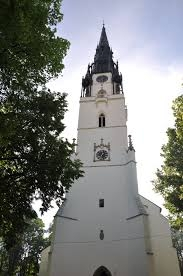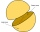# Castle tower

The castle tower has a cone-shaped roof with a diameter of 10 meters and a height of 8 meters. Calculate how much m² of coverage is needed to cover it if we must add one-third for the overlap.

Result

S =  197.585 m2

#### Solution:

$D=10 \ \text{m} \ \\ h=8 \ \text{m} \ \\ \ \\ r=D/2=10/2=5 \ \text{m} \ \\ \ \\ s=\sqrt{ h^2 + r^2 }=\sqrt{ 8^2 + 5^2 } \doteq \sqrt{ 89 } \ \text{m} \doteq 9.434 \ \text{m} \ \\ \ \\ S_{1}=\pi \cdot \ r \cdot \ s=3.1416 \cdot \ 5 \cdot \ 9.434 \doteq 148.1886 \ \text{m}^2 \ \\ \ \\ S=(1+\dfrac{ 1 }{ 3 } ) \cdot \ S_{1}=(1+\dfrac{ 1 }{ 3 } ) \cdot \ 148.1886 \doteq 197.5848 \doteq 197.585 \ \text{m}^2$Our examples were largely sent or created by pupils and students themselves. Therefore, we would be pleased if you could send us any errors you found, spelling mistakes, or rephasing the example. Thank you!

Leave us a comment of this math problem and its solution (i.e. if it is still somewhat unclear...):Be the first to comment!Tips to related online calculators
Need help calculate sum, simplify or multiply fractions? Try our fraction calculator.
Pythagorean theorem is the base for the right triangle calculator.

## Next similar math problems:

1. Observation towerFrom the observation tower at a height of 105 m above sea level, the ship is aimed at a depth angle of 1° 49´. How far is the ship from the base of the tower?Calculate the surface of a quadrilateral pyramid, which has a rectangular base with dimensions a = 8 cm, b = 6 cm and height H = 10 cm.
3. Two hemispheresIn a wooden hemisphere with a radius r = 1, a hemispherical depression with a radius r/2 was created so that the bases of both hemispheres lie in the same plane. What is the surface of the created body (including the surface of the depression)?
4. Concentric circles and chordIn a circle with a diameter d = 10 cm, a chord with a length of 6 cm is constructed. What radius have the concentric circle while touch this chord?
5. Closed circuitIn a closed circuit, there is a voltage source with U1 = 12 V and with an internal resistance R1 = 0.2 Ω. The external resistance is R2 = 19.8 Ω. Determine the electric current and terminal voltage.
6. Side lengthsIn the triangle ABC, the height to the side a is 6cm. The height to side b is equal to 9 cm. Side "a" is 4 cm longer than side "b". Calculate the side lengths a, b.
7. Pentagonal prismThe regular pentagonal prism is 10 cm high. The radius of the circle of the described base is 8 cm. Calculate the volume and surface area of the prism.
8. The right triangleThe right triangle ABC has a leg a = 36 cm and an area S = 540 cm2. Calculate the length of the leg b and the median t2 to side b.
9. Wire fenceThe wire fence around the garden is 160 m long. One side of the garden is three times longer than the other. How many meters do the individual sides of the garden measure?
10. Slow saving in banksHow long will it take to save € 9,000 by depositing € 200 at the beginning of each year at 2% interest?
11. What isWhat is the annual percentage increase in the city when the population has tripled in 20 years?
12. Age of familyThe age of father and son is in the ratio 10: 3. The age of the father and daughter is in ratio 5: 2. How old are a father and a son if the daughter is 20?
13. Viewing angleThe observer sees a straight fence 60 m long at a viewing angle of 30°. It is 102 m away from one end of the enclosure. How far is the observer from the other end of the enclosure?
14. Triangle from medianCalculate the perimeter, content, and magnitudes of the remaining angles of triangle ABC, given: a = 8.4; β = 105° 35 '; and median ta = 12.5.
15. Interior anglesCalculate the interior angles of a triangle that are in the ratio 2: 3: 4.
16. The farmerThe farmer calculated that the supply of fodder for his 20 cows was enough for 60 days. He decided to sell 2 cows and a third of the feed. How long will the feed for the rest of the peasant's herd last?
17. In aIn a triangle, the aspect ratio a: c is 3: 2 and a: b 5: 4. The perimeter of the triangle is 74cm. Calculate the lengths of the individual sides.